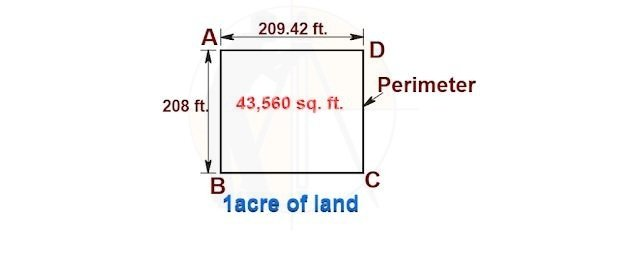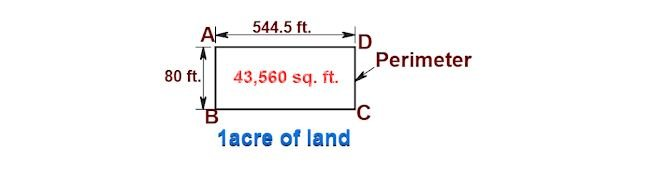Breaking News
Home / Civil Engineering / What Will Be The Cost Of Fencing Per Acre ?

# What Will Be The Cost Of Fencing Per Acre ?

## What Will Be The Cost Of Fencing Per Acre ?

Now, let us find out the answer for one of the FAQs “What will be the cost of fencing per acre?”

My response is, This is a non-answerable wrong question.

The reason is, the area of any land does not define its perimeter. The cost differs from one land to another having the same measured area.

I will illustrate this with the following 2 examples.

1. Land no 1:Given data:

Length of  side AB = CD = 208 ft.

Length of side AD = BC = 209.42 ft.

Area of rectangular land

= [209.42 ft. × 208 ft.]

= 43,560 sq ft. = 1 acre

[ As 1acre = 43,560 sq ft.]

Perimeter of land no 1.

= [side AB + side BC + side CD + side DA]

= [208 ft. + 209.42 ft. + 208 ft. + 209.42 ft.]

834.84 ft.

2. Land no 2:Given data:

Length of  side AB = CD = 80 ft.

Length of side AD = BC = 544.5 ft.

Area of rectangular land

= [544.5 ft. × 80 ft.]

= 43,560 sq ft. = 1 acre

[ As 1acre = 43,560 sq ft.]

The perimeter of land no 2.

= [side AB + side BC + side CD + side DA]

= [80 ft. + 544.5 ft. + 80 ft. + 544.5 ft.]

1249 ft.

### Basic Components of Building Structure | Building Elements | Sub Structure | Super Structure

Now, let us consider installing a barbed-wire fence of the same height for both types of land.

Estimation & costing of barbed wire fencing for land./ Calculating the cost of barbed wire fencing for the land.

(part 4).

Let us find out the material & labor cost for barbed wire fencing for the land.

Material cost:

Sl.No.   Description        Quantity      Rate      Unit      Amount

1.    RCC fencing poles     40.0           270         no.     10,800=00

2.   Boulder for packing  0.42417    1400       cum        593=83

3.    Cement bags for

concreting                 6.837         400        bag      2734=80

4.    Sand for

concreting                0.3563       2200       cum.     783=86

5.    Aggregate for

concreting                   0.4751         1850       cum.     878=93

6.   Barbed wire for

fencing                      37.88             150           kg.     5682=00

Total material cost for fencing =  INR  21,473.42

Labor cost:

Sl.No.   Description        Quantity      Rate      Unit      Amount

1.   Earthwork excavation

for the fencing pole    1.27251          800    cum         1017=60

2.    Installation and

concreting the pole         40              120      no.          4800=00

3.  Tying fencing wire        840                3      meter      2520=00

Total labor cost for fencing =INR  8337.00

The total cost of fencing the land

= [total material cost + labor cost]

= [ 21473.42 + 8337]

INR  29810.42

### What is Gravity Dam?

Cost per running ft.

= [total cost ÷ perimeter of the land in ft.]

= [29810.42 ÷ 260 ft.]

=  114.65/R.FT.

= 376.64 / running meter.

1. Cost of fencing for land 1

= [ perimeter  × fence cost/ r ft.]

= [ 834.84 ft.  × 114.65/-]

INR 95,714.4/-

2. Cost of fencing for land 2

= [ perimeter  × fence cost/ r ft.]

= [ 1249 ft.  × 114.65/-]

= INR 1,43,197.85/-

Note: The fencing cost / r ft. is taken from the above-linked article.

The difference in the fencing cost of land 1 & land 2

= [1,43,197.85 – 95, 714.4]

47,483.45/-

i.e. whopping 49.6% difference in the fencing cost of the 1-acre of land having different dimensions.
I hope you understood why we cannot answer this question.

## . Land Surveying & Architects

### THANKS.